# MATLAB之基本语法与基础函数

## 常用命令

dir：列出当前目录下的所有文件

clc：清除命令窗

clear all：清除环境（从内存中清除所有变量）

who：将内存中的当前变量以简单形式列出

close all: 关闭所有的 Figure 窗口

## 变量设置

(1).变量命名

MATLAB 的变量名以字母打头，后最多可跟 19 个字母或数字，如 x,y,ae3 和  d3er45  等都是合法的变量名，不能使用内部函数或命令名作为变量名。

MATLAB 中的变量名区分大小，ab 与  Ab 表示两个不同的变量。

(2).变量赋值

l     表达式赋值

>>a=(100*0.02)/4.0

a=0.0500

l     矩阵赋值

>> C=[-1,0,0;1,-1,0;0,0,2]；(省略最后的分号，MATLAB  会回显矩阵值)

l     通过引用特定的位置可以单独改变某个矩阵元素

l     可以引用已定义的矩阵，重新定义一个新矩阵。

>>B=[3 S 2]

B=35642

## 整数操作

l     fix(x)：截尾取整

>> fix( [3.12 -3.12])

ans =3 -3

l     floor(x)：不超过 x 的最大整数(高斯取整)

>> floor( [3.12 -3.12])

ans =3 -4

l     ceil(x) ：大于 x 的最小整数

>> ceil( [3.12 -3.12])

ans =4 -3

## 随机序列常用命令

l     rand：均匀分布随机矩阵

rand                                   %无变量输入时只产生一个随机数

y= rand(n)                         %生成 n×n 随机矩阵，其元素在（0，1）内

y = rand(m,n)                    %生成 m×n 随机矩阵，其元素在（0，1）内

l     randn:正态分布随机矩阵

randn                                    %无变量输入时只产生一个正态分布随机数

y = randn(n)                         %生成 n×n 正态分布随机矩阵

y = randn(m,n)                     %生成 m×n 正态分布随机矩阵

MATLAB 命令为：>> mu=0.6；sigma=0.1；>> x=mu+sqrt(sigma)*randn(4)。

l     randsrc：产生均匀分布数组

randsrc                                  %无变量输入时只产生一个随机数 1 或者-1

y= randsrc(n)                         %生成 n×n 随机数组，其元素为 1 或者-1

y = randsrc(m,n)                    %生成 m×n 随机数组，其元素为 1 或者-1

## 矩阵常用操作命令

MATLAB  的基本单位是矩阵，掌握矩阵的输入、各种数值运算以及矩阵函

(1).矩阵的输入

l     直接输入创建矩阵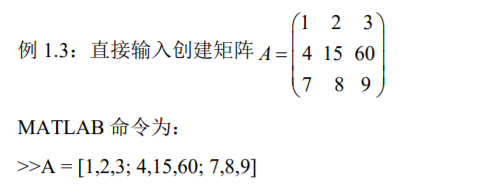l     用矩阵函数来生成矩阵

MATLAB  提供了大量的函数来创建特殊矩阵，表 1.1 给出 MATLAB 常用的矩阵函数。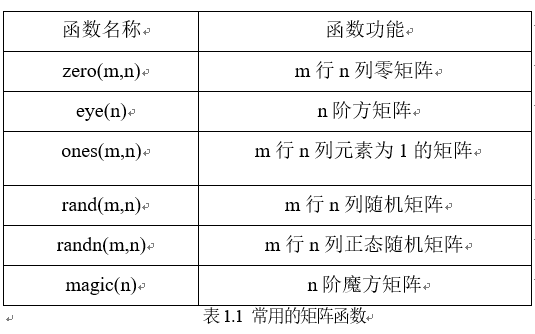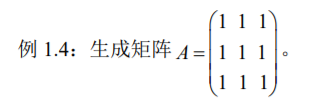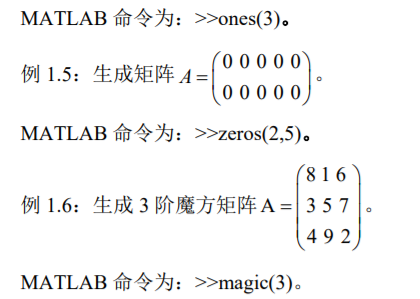(2).操作符“：”的说明

j:k表示步长为 1 的等差数列构成的数组：[j, j+1, j+2,…, k]。

j:i:k表示步长为 i 的等差数列构成的数组：[j,j+i,j+2*i,…, k]。

A(i:j)表示 A(i)，A(i+1)，…，A(j)。

(3).对矩阵元素的操作

A(i,j)表示矩阵 A 的第 i 行第 j 列元素。

A(:,j)表示矩阵 A 的第 j 列。

A(i,:)表示矩阵 A 的第 i 行。

A(:,:)表示 A 的所有元素构造 2 维矩阵

A(:)表示以矩阵 A 的所有元素按列构成的一个列矩阵。

A(i)表示矩阵 A(:)的第 i 个元素。

[]表示空矩阵。

(4).矩阵的运算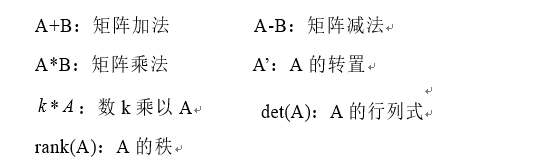(5).数组

l     特殊数组的创建

linspace(a,b,n) 给出区间[a,b]的 n 个等分点数据

MATLAB 命令为：>> linspace(0,1,6)

l     数组运算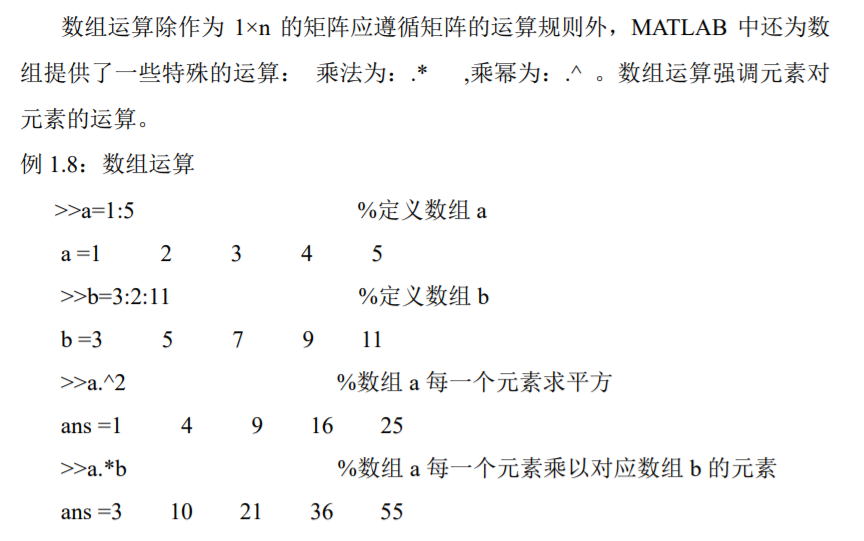## 位操作

l     bitand：按位与

C=bitand(A,B)命令将返回两个非负整数数组 A 和 B 的相应元素按位与操作的结果。为了确保 A 和 B 的元素都是整数，可以使用 ceil、fix、floor 和 round函数来生成 A 和 B。

l     bitor：按位或

C=bitor(A,B)命令将返回两个非负整数数组 A 和 B 的相应元素按位或操作的结果。为了确保 A 和 B 的元素都是整数，可以使用 ceil、fix、floor 和 round 函数来生成 A 和 B。

l     bitxor：按位异或

C=bitxor(A,B)返回两个非负整数数组 A 和 B 的相应元素进行按位异或的结果，为了确保 A 和 B 的元素都是整数，可以使用 ceil、fix、floor 和 round 函数来生成 A 和 B。

l     bitset：设置指定位的值

C=bitset(A,bit,v)命令将 A 中元素第 bit 位设为 v，其中 v 必须为 0 或 1，A 中的元素必须为非负整数，bit 必须为 1 到 A 中元素浮点整数表示法的位数之间的一个数字。

l     bitget：获取指定位的值

C=bitget(A,bit)命令将返回 A 中元素用 bit 指定位的值，A 中的元素必须为非负整数，bit 必须为 1 到 A 中元素浮点整数表示法位数之间的一个数字。

>>C=bitget(1,2)

## 绘图操作

（1）图形标注

title(‘string’,’属性名’,’属性值’,…) ——  给图形加标题

xlabel (‘string’,’属性名’,’属性值’,…) ——  给 x 轴加标注

ylabel (‘string’,’属性名’,’属性值’,…) ——  给 y 轴加标注

legend (‘string1’,’string2’,…)——  添加图例，其顺序对应于绘图指令中的顺序

axis ([xmin,xmax,ymin,ymax])——  控制坐标轴的刻度范围

（2）二维图形

l     plot(x,y)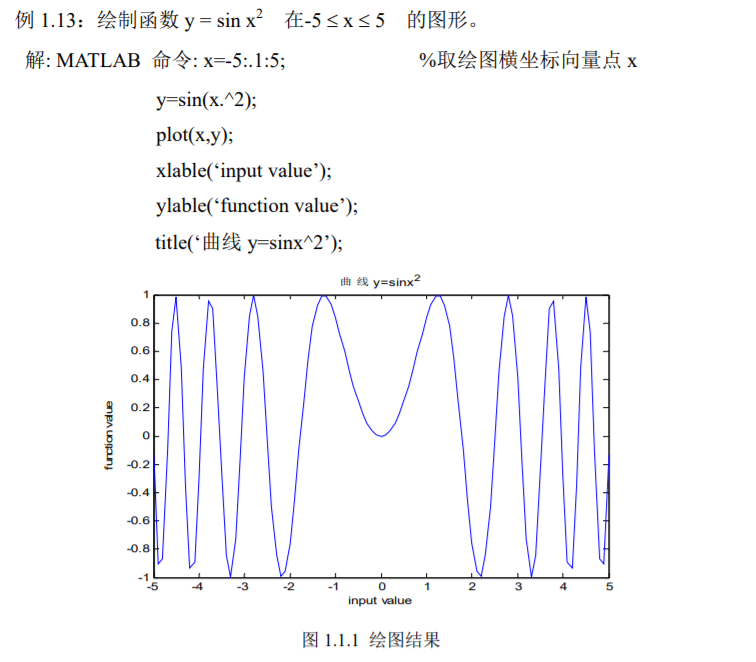l     plot(x1,y1,x2,y2,x3,y3…)

y1=f(x1),y2=f(x2),y3=f(x3)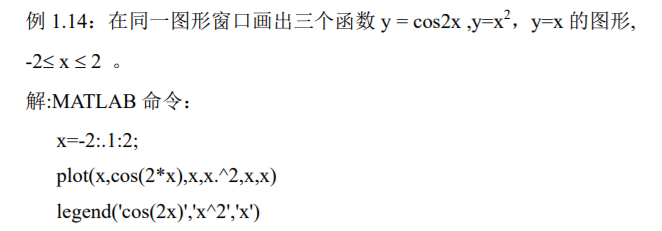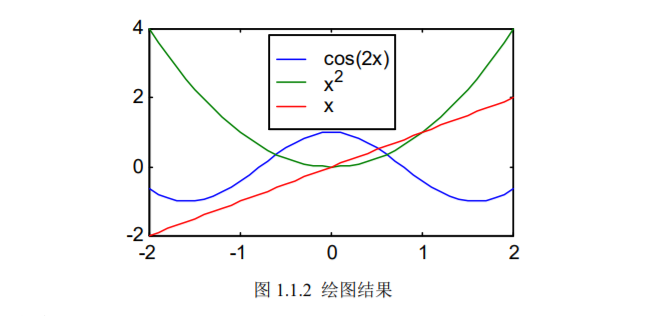(3)二维特殊图形

l     bar

>> x=1:5;

>> bar(x),title('直方图')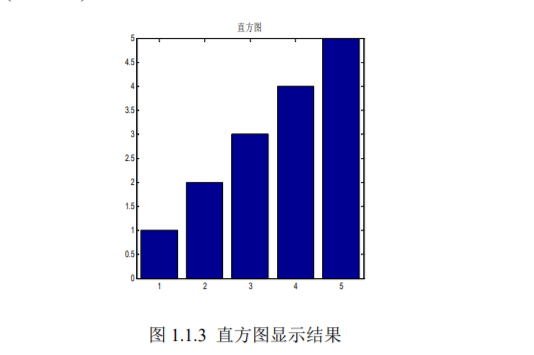l     Hist 表示生成直方图

M=hist(N)表示将 N 中的最大最小值找出来，然后，平均取十个等间隔点，看以每个间隔点为中心，向两边各扩展 1/2 间隔的范围内，包括 N 的元素个数，因此 M 返回值都是 1*10 大小。

>>M=hist(b2)

>>M=hist(b1)

## 文件操作

1fopen  打开文件

fopen 函数的调用格式为：fid= fopen(文件名，打开方式)

2  fclose  关闭文件

3）  二进制文件的读写操作

l     fwrite  写二进制文件

fwrite  函数按照指定的数据类型将矩阵中的元素写入到文件中。其调用格式为：COUNT=fwrite  (fid,  A,  precision)，其中 COUNT 返回所写的数据元素个数，fid为文件句柄，A 用来存放写入文件的数据，precision 用于控制所写数据的类型，其形式与 fread 函数相同。

## M 文件的建立与使用

M 文件有命令文件和函数文件两种形式，这两种文件的扩展名相同，都是.m。当用户要运行的命令较多时，直接从键盘上逐条输入较为繁琐，可利用命令文件来解决多行输入问题。用户可将一组相关命令编辑在同一个命令文件中，运行时只需输入文件名字，MATLAB  就会自动按顺序执行文件中的命令。函数文件是另一种形式的 M 文件，它的第一句可执行语句是以 function 引导的定义语句，在函数文件中的变量都是局部变量。

1）命令文件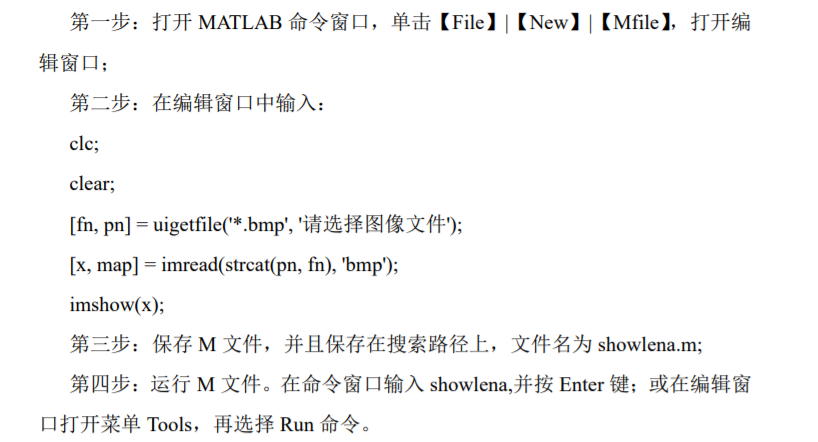（2）函数文件

M 函数文件的一般形式为：function <因变量>=<函数名>(<自变量>)

M 函数文件可以有多个因变量和多个自变量，当有多个因变量时用[]括起来。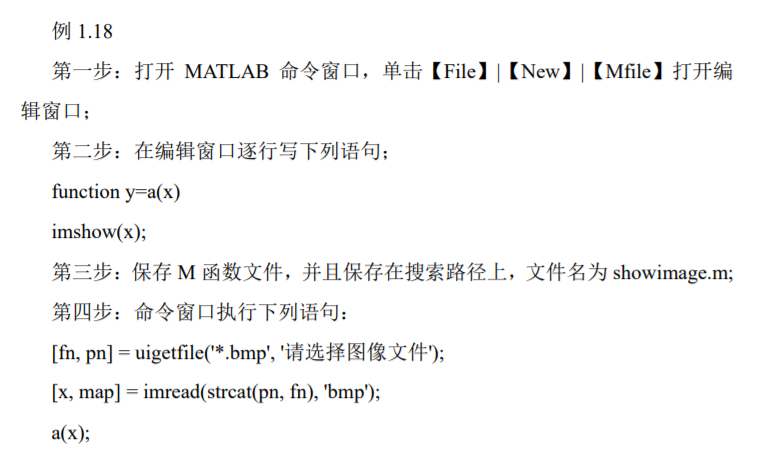posted @ 2019-09-12 00:46  Qftm  阅读(653)  评论(0编辑  收藏